SSC CGL Previous Year Questions: Mensuration - 2

# SSC CGL Previous Year Questions: Mensuration - 2 - SSC CGL

Test Description

## 30 Questions MCQ Test SSC CGL (Tier - 1) - Previous Year Papers (Topic Wise) - SSC CGL Previous Year Questions: Mensuration - 2

SSC CGL Previous Year Questions: Mensuration - 2 for SSC CGL 2023 is part of SSC CGL (Tier - 1) - Previous Year Papers (Topic Wise) preparation. The SSC CGL Previous Year Questions: Mensuration - 2 questions and answers have been prepared according to the SSC CGL exam syllabus.The SSC CGL Previous Year Questions: Mensuration - 2 MCQs are made for SSC CGL 2023 Exam. Find important definitions, questions, notes, meanings, examples, exercises, MCQs and online tests for SSC CGL Previous Year Questions: Mensuration - 2 below.
Solutions of SSC CGL Previous Year Questions: Mensuration - 2 questions in English are available as part of our SSC CGL (Tier - 1) - Previous Year Papers (Topic Wise) for SSC CGL & SSC CGL Previous Year Questions: Mensuration - 2 solutions in Hindi for SSC CGL (Tier - 1) - Previous Year Papers (Topic Wise) course. Download more important topics, notes, lectures and mock test series for SSC CGL Exam by signing up for free. Attempt SSC CGL Previous Year Questions: Mensuration - 2 | 30 questions in 30 minutes | Mock test for SSC CGL preparation | Free important questions MCQ to study SSC CGL (Tier - 1) - Previous Year Papers (Topic Wise) for SSC CGL Exam | Download free PDF with solutions
 1 Crore+ students have signed up on EduRev. Have you?
SSC CGL Previous Year Questions: Mensuration - 2 - Question 1

### Three solid spheres of radius 3 cm, 4 cm, and 5 cm are melted and recasted into a solid sphere. What will be the percentage decrease in the surface area?    (SSC CGL 2017)

Detailed Solution for SSC CGL Previous Year Questions: Mensuration - 2 - Question 1

Total surface area of three solid spheres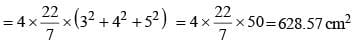Now,
Volume of new sphere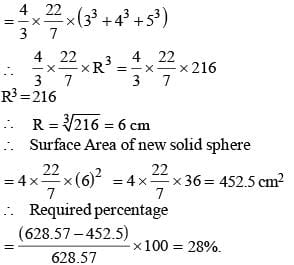SSC CGL Previous Year Questions: Mensuration - 2 - Question 2

### If the diameter of a sphere is 14 cm, then what is the surface area (in cm2) of the sphere?   (SSC CGL 2017)

Detailed Solution for SSC CGL Previous Year Questions: Mensuration - 2 - Question 2

Diameter of sphere = 14 cm
∴ radius = 14/2 = 7 cm
∴ Surface area of sphere = 4 πr2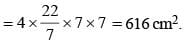SSC CGL Previous Year Questions: Mensuration - 2 - Question 3

### A solid sphere of diameter 17.5 cm is cut into two equal halves. What will be the increase (in cm2) in the total surface area?    (SSC CGL 2017)

Detailed Solution for SSC CGL Previous Year Questions: Mensuration - 2 - Question 3

Here,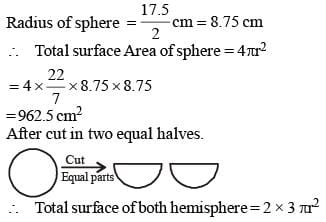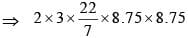⇒ 1443.75 cm2
∴ Required increased area = (1443.75 – 962.5)
=  481.25 cm2

SSC CGL Previous Year Questions: Mensuration - 2 - Question 4

A cylindrical rod of radius 30 cm and length 40 cm is melted and made into spherical balls of radius 1 cm. The number of spherical balls is    (SSC Sub. Ins. 1st Sit. 2015)

Detailed Solution for SSC CGL Previous Year Questions: Mensuration - 2 - Question 4

Total number of spherical balls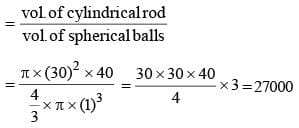SSC CGL Previous Year Questions: Mensuration - 2 - Question 5

The radii of the base of a cylinder and a cone are equal and their volumes are also equal. Then the ratio of their heights is    (SSC Sub. Ins. 1st Sit. 2015)

Detailed Solution for SSC CGL Previous Year Questions: Mensuration - 2 - Question 5

Vol. of cylinder = Vol. of cone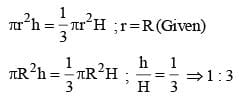SSC CGL Previous Year Questions: Mensuration - 2 - Question 6

The curved surface area of a cylinder with its height equal to the radius, is equal to the curved surface area of a sphere.
The ratio of volume of the cylinder to that of the sphere is    (SSC Sub. Ins. 1st Sit. 2015)

Detailed Solution for SSC CGL Previous Year Questions: Mensuration - 2 - Question 6

Ler r be the radius of cylinder and r' be the radius of the sphere.
CSA of cylinder = 2πrh
2πr2 (∴ r = h)
CSA of sphere = 4πr2
ATQ
CSA of cylinder = CSA of sphere
= 2πr2 = 4πr'2
⇒ r2 = 2r'2
Now,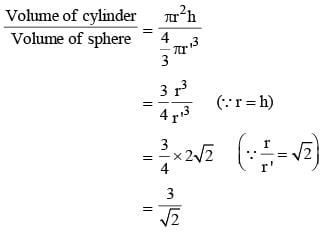SSC CGL Previous Year Questions: Mensuration - 2 - Question 7

The radius and the height of a cone are each increased by 20%. Then the volume of the cone increases by    (SSC CGL  1st Sit. 2016)

Detailed Solution for SSC CGL Previous Year Questions: Mensuration - 2 - Question 7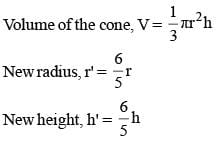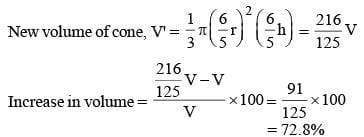SSC CGL Previous Year Questions: Mensuration - 2 - Question 8

A solid sphere of radius 9 cm is melted to form a sphere of radius 6 cm and a right circular cylinder of same radius. The height of the cylinder so formed is    (SSC CGL 1st Sit. 2016)

Detailed Solution for SSC CGL Previous Year Questions: Mensuration - 2 - Question 8

Radius of large sphere = 9 cm
Radius of smaller sphere = 6 cm
Radius of cylinder = 6 cm
Height of cylinder = h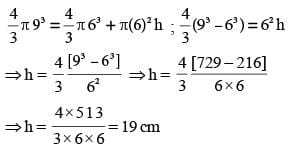SSC CGL Previous Year Questions: Mensuration - 2 - Question 9

The base area of a right pyramid is 57 sq. units and height is 10 units. Then the volume of the pyramid is    (SSC CGL  1st Sit. 2016)

Detailed Solution for SSC CGL Previous Year Questions: Mensuration - 2 - Question 9

Volume of Pyramid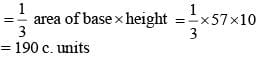SSC CGL Previous Year Questions: Mensuration - 2 - Question 10

The area of the largest sphere (in cm2) that can be drawn inside a square of side 18 cm is    (SSC CGL  1st Sit. 2016)

Detailed Solution for SSC CGL Previous Year Questions: Mensuration - 2 - Question 10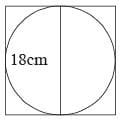Side of square = Diameter of sphere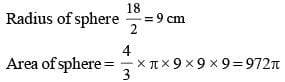SSC CGL Previous Year Questions: Mensuration - 2 - Question 11

5 persons will live in a tent. If each person requires 16m2 of floor area and 100 m3 space for air then the height of the cone of smallest size to accommodate these persons would be?    (SSC CGL 1st Sit. 2015)

Detailed Solution for SSC CGL Previous Year Questions: Mensuration - 2 - Question 11

For surface Area of 5 persen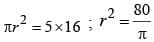Now, volume of cone = volume of air space of 5 person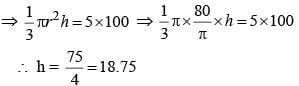SSC CGL Previous Year Questions: Mensuration - 2 - Question 12

If the volume of a sphere is numerically equal to its surface area then its diameter is:    (SSC CGL 1st Sit. 2015)

Detailed Solution for SSC CGL Previous Year Questions: Mensuration - 2 - Question 12

According to question,
Volume of sphere= surface area of sphere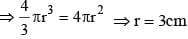Diameter = 6 cm

SSC CGL Previous Year Questions: Mensuration - 2 - Question 13

A square is inscribed in a quarter–circle in such a manner that two of its adjacent vertices lie on the two radii at an equal distance from the centre, while the other two vertices lie on the circular arc. If the square has sides of length x, then the radius of the circle is    (SSC CGL 1st Sit. 2015)

Detailed Solution for SSC CGL Previous Year Questions: Mensuration - 2 - Question 13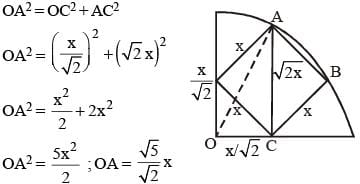SSC CGL Previous Year Questions: Mensuration - 2 - Question 14

If the area of a circle is A, radius of the circle is r and circumference of it is C, then    (SSC CGL 1st Sit. 2015)

Detailed Solution for SSC CGL Previous Year Questions: Mensuration - 2 - Question 14

Area of circle, A = πr2 ...(i)
Circumference of circle, C = 2πr ...(ii)
Multiplying eq. (i) by 2, we get, 2A = 2πr2
Multiplying eq. (ii), by ‘r’, we get rC = 2πr2
∴ rC = 2A

SSC CGL Previous Year Questions: Mensuration - 2 - Question 15

The perimeter of one face of a cube is 20 cm. Its volume will be    (SSC CGL 1st Sit. 2015)

Detailed Solution for SSC CGL Previous Year Questions: Mensuration - 2 - Question 15

Perimeter of one face, 4a = 20 cm
Therefore, side of cube, a = 5 cm
Volume of cube= a3 = 53 = 125 cm3

SSC CGL Previous Year Questions: Mensuration - 2 - Question 16

The surface area of a sphere is 616 cm2. The volume of the sphere would be:    (SSC CHSL 2015)

Detailed Solution for SSC CGL Previous Year Questions: Mensuration - 2 - Question 16

Surface area of sphere = 616 cm2
4 πr2 = 616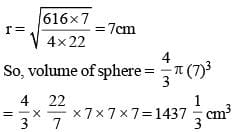SSC CGL Previous Year Questions: Mensuration - 2 - Question 17

The outer circumference of a circular race–track is 528 metre. The track is everywhere 14 metre wide. Cost of levelling the track at the rate of ₹ 10 per sq. metre is:    (SSC CHSL 2015)

Detailed Solution for SSC CGL Previous Year Questions: Mensuration - 2 - Question 17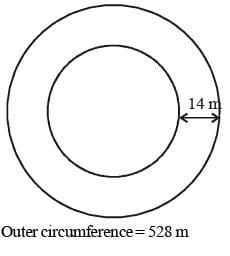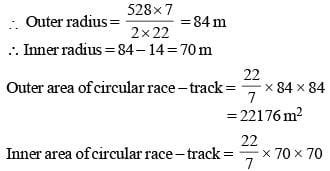= 15400 m2.
So area of track = 22176 – 15400 = 6776 m2
Cost of levelling the circular track = 6776 × 10 = ₹ 67760

SSC CGL Previous Year Questions: Mensuration - 2 - Question 18

The volume of the largest right circular cone that can be cut out of a cube of edge 7 cm? (Use π = 22/7)      (SSC CHSL 2015)

Detailed Solution for SSC CGL Previous Year Questions: Mensuration - 2 - Question 18

Volume of right circular cone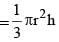Radius of cone = 3.5 cm
Height of cone = 7cm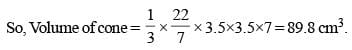SSC CGL Previous Year Questions: Mensuration - 2 - Question 19

If water is freezed to become ice, its volume is increased by 10%, then if the ice is melted to water again, its volume will be decreased by:    (SSC CHSL 2015)

Detailed Solution for SSC CGL Previous Year Questions: Mensuration - 2 - Question 19

Let initial volume = 100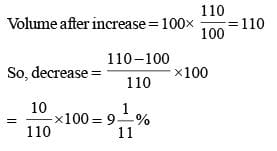SSC CGL Previous Year Questions: Mensuration - 2 - Question 20

A hemispherical bowl has internal radius of 6 cm. The internal surface area  would be: (take π = 3.14)    (SSC CHSL 2015)

Detailed Solution for SSC CGL Previous Year Questions: Mensuration - 2 - Question 20

Internal Radius of hemisphere = 6 cm
Internal surface area = 2πr2
= 2 × 3.14 × (6)2
= 226.08 cm2.

SSC CGL Previous Year Questions: Mensuration - 2 - Question 21

The length of two parallel sides of a trapezium are 15 cm and 20 cm.  If its area is 175 sq. cm, then its height is:    (SSC CHSL 2015)

Detailed Solution for SSC CGL Previous Year Questions: Mensuration - 2 - Question 21

Area of trapezium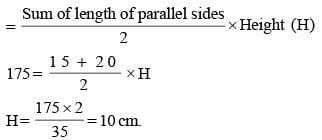SSC CGL Previous Year Questions: Mensuration - 2 - Question 22

If the base of right prism remains same and the measures of the lateral edges are halved, then its volume will be reduced by:    (SSC Sub. Ins. 2015)

Detailed Solution for SSC CGL Previous Year Questions: Mensuration - 2 - Question 22

Volume of prism = base area ×  height = A × h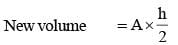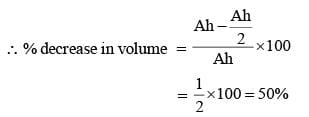SSC CGL Previous Year Questions: Mensuration - 2 - Question 23

The perimeter of a sheet of paper in the shape of a quadrant of a circle is 75 cm. Its area would be  (π = 22/7)    (SSC Sub. Ins. 2015)

Detailed Solution for SSC CGL Previous Year Questions: Mensuration - 2 - Question 23

Perimeter of quadrant of a circle = 75 cm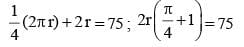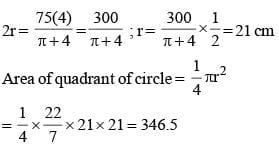SSC CGL Previous Year Questions: Mensuration - 2 - Question 24

The total surface area of a regular triangular pyramid with each edges of length 1cm is:    (SSC Sub. Ins. 2015)

Detailed Solution for SSC CGL Previous Year Questions: Mensuration - 2 - Question 24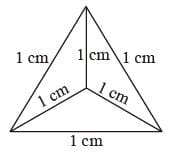Regular equilateral triangular pyramid Total surface area of pyramid = 4 × Area of Equilateral triangle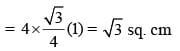SSC CGL Previous Year Questions: Mensuration - 2 - Question 25

A vessel full of pure acid contains 10 litres of it, of which 2 litres are withdrawn. The vessel is then filled with water. Next 2 litres of the mixture are withdrawn, and again the vessel is filled up with water. The ratio of the acid left in the vessel with that of the original quantity is    (SSC CGL 2014)

Detailed Solution for SSC CGL Previous Year Questions: Mensuration - 2 - Question 25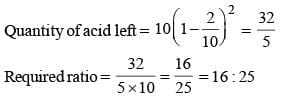SSC CGL Previous Year Questions: Mensuration - 2 - Question 26

A wire is bent into the form of a circle, whose area is 154 cm2. If the same wire is bent into the form of an equilateral triangle, the approximate area of the equilateral triangle is    (SSC CGL  2014)

Detailed Solution for SSC CGL Previous Year Questions: Mensuration - 2 - Question 26

Let r be the radius of circle.
Area of the wire = 154 cm2
πr2 = 154 cm2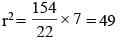r = 7 cm
length of wire = circumference of circle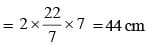Now, perimeter of equilateral triangle = 44 cm side =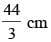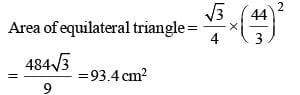SSC CGL Previous Year Questions: Mensuration - 2 - Question 27

There are two cones. The curved surface area of one is twice that of the other. The slant height of the latter is twice that of the former. The ratio of their radii is    (SSC CGL 2014)

Detailed Solution for SSC CGL Previous Year Questions: Mensuration - 2 - Question 27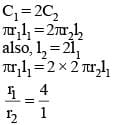SSC CGL Previous Year Questions: Mensuration - 2 - Question 28

The base of a right pyramid is an equilateral triangle of side 4 cm each. Each slant edge is 5 cm long. The volume of the pyramid is    (SSC CGL 2014)

Detailed Solution for SSC CGL Previous Year Questions: Mensuration - 2 - Question 28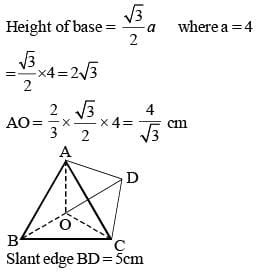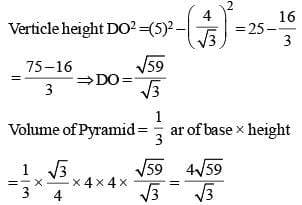SSC CGL Previous Year Questions: Mensuration - 2 - Question 29

The external fencing of a circular path around a circular plot of land is 33 m more than its interior fencing. The width of the path around the plot is    (SSC CHSL 2014)

Detailed Solution for SSC CGL Previous Year Questions: Mensuration - 2 - Question 29

Let radius of internal and external circular Plot be r and R respectively.
2πR – 2πr  = 33 m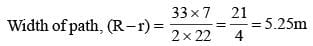SSC CGL Previous Year Questions: Mensuration - 2 - Question 30

The area of a square park is 25 sq. km. The time taken to complete a round of the field once, at a speed of 3 km/hour is    (SSC CHSL 2014)

Detailed Solution for SSC CGL Previous Year Questions: Mensuration - 2 - Question 30

Side of square park = √25 km = 5 km
Perimeter of park = 4 × 5 = 20 km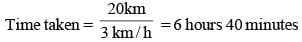## SSC CGL (Tier - 1) - Previous Year Papers (Topic Wise)

250 tests
Information about SSC CGL Previous Year Questions: Mensuration - 2 Page
In this test you can find the Exam questions for SSC CGL Previous Year Questions: Mensuration - 2 solved & explained in the simplest way possible. Besides giving Questions and answers for SSC CGL Previous Year Questions: Mensuration - 2, EduRev gives you an ample number of Online tests for practice

250 tests